Mobile QR Code1. (Department of Electronic and Electrical Engineering, Pohang University of Science and Technology, Pohang 37673, Korea)
2. (Department of Electrical Engineering, Rämistrasse 101, 8092 Zürich, Switzerland)
3. (Department of Electronic and Electrical Engineering, Pohang University of Science and Technology, Pohang 37673, Korea)

Temperature sensor, subthreshold, bandgap reference

## I. INTRODUCTION

Ultra-low-power (ULP) sensor applications often necessitate a continuous monitoring of temperature sensor. It is not only to obtain the accurate sensor output by calibrating the measurement results but also to compensate strong temperature sensitivity of circuit operation. For a continuous thermal monitoring block to work with a nW-consuming sensor node, the power consumption needs to be even lower, e.g. in sub-nW level. However, extreme reduction of the power consumption eventually faces challenges in using analog circuits which require a nonzero bias current.

On-chip temperature sensing schemes utilize inherent temperature dependence of devices provided in CMOS technology. Key coefficients of complementary-to-absolute-temperature (CTAT) and proportional-to-absolute-temperature (PTAT) can be obtained from CMOS compatible circuits and converted through an analog-to-digital (AD) conversion. Using BJT devices in temperature sensing references the bandgap voltage (V$_{\mathrm{BG}}$) which shows superiority in robustness against process variations (1-5). It is because the temperature coefficients of CTAT and PTAT are well-defined by inherent PN junction characteristic and design parameters, respectively. However, previous works use a high-precision oversampling ADC that dissipates a significant power of ${μ}$W range (1-5). As an alternative, the use of a temperature-dependent frequency generation followed by an edge counting is one of the most popular ways in ULP applications (6-12) since an oscillator circuit can be designed to consume a low power and can be implemented in a small area. This approach suffers from nonlinear die-to-die variations and necessitates a 2-point calibration. In addition, the temperature coefficient of the oscillator frequency is very low and takes a long conversion time for the edge counting. To further reduce power consumption of the on-chip temperature sensor, a leakage-based temperature-sensing circuits was combined with a Nyquist-rate asynchronous successive approximation register (SAR) ADC (13). Though it achieved the lowest power consumption of 490 pW, the minimum temperature resolution given by the 1-b resolution of the ADC is about 0.6 $^{\circ}$C.

This paper presents a ULP temperature sensor based on a leakage-based reference generator (14). A reference divider with a switched-capacitor circuit effectively achieves a finer resolution of SAR ADC. The internal nodes of the reference circuit are directly sampled to be passed to the ADC. It minimizes the use of analog circuits which necessitate bias current. The designed temperature sensor is implemented using 180 nm CMOS process. It achieves a 62.6-pJ/conversion with a resolution of 0.37 $^{\circ}$C. Section II describes circuits of the proposed temperature sensor, and Section III shows implementation results. Section IV concludes this work.

Fig. 1. Overall circuit diagram of the proposed temperature sensor (*SMP : SAMPLE).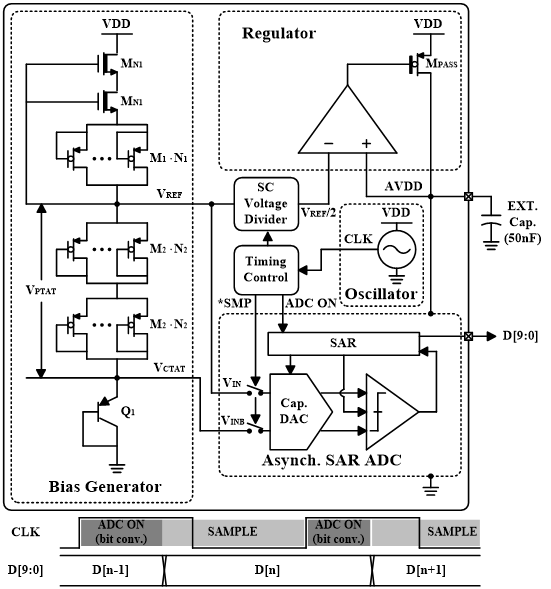## II. CIRCUIT DESCRIPTION

Fig. 1 shows an overall circuit diagram of the proposed temperature sensor. The sensor consists of a bias generator part, a regulator part, an asynchronous SAR ADC, a switched-capacitor (SC) voltage divider and a timing control block with an oscillator. The regulator is formed with a conventional differential amplifier (Fig. 2). Since the bias current, I$_{\mathrm{B}}$, is in a pA level, a large output resistance associated at OUT node causes a pole at a low frequency. However, since AVDD is the supply voltage for ADC, AVDD node is desired to be the dominant pole with a large power-decoupling capacitance connected at AVDD. We connected an off-chip capacitor of 50 nF at AVDD node to make it the dominant pole in the regulator loop.

The bias generator is based on the leakage-based hybrid V$_{\mathrm{BG}}$-V$_{\mathrm{TH}}$ voltage reference (14) which provides a PTAT (V$_{\mathrm{PTAT}}$), a CTAT (V$_{\mathrm{CTAT}}$) and a reference voltage (V$_{\mathrm{REF}}$). The V$_{\mathrm{REF}}$ is divided by half using SC voltage divider. The reduced supply voltage by the SC voltage divider decreases the input conversion range of ADC and effectively achieves a finer temperature resolution. With the 180 nm CMOS process used in this work, the V$_{\mathrm{REF}}$ and the AVDD is about 0.8 V and 0.4 V, respectively. A static voltage divider using resistors should flow a nonzero static current.

Fig. 2. Amplifier circuit in the regulator and DC characteristics.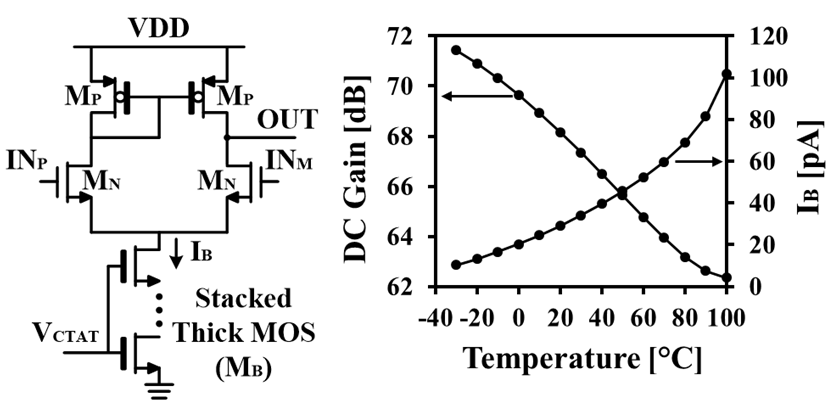Fig. 3. (a) SC voltage divider circuit, (b) simulated transients.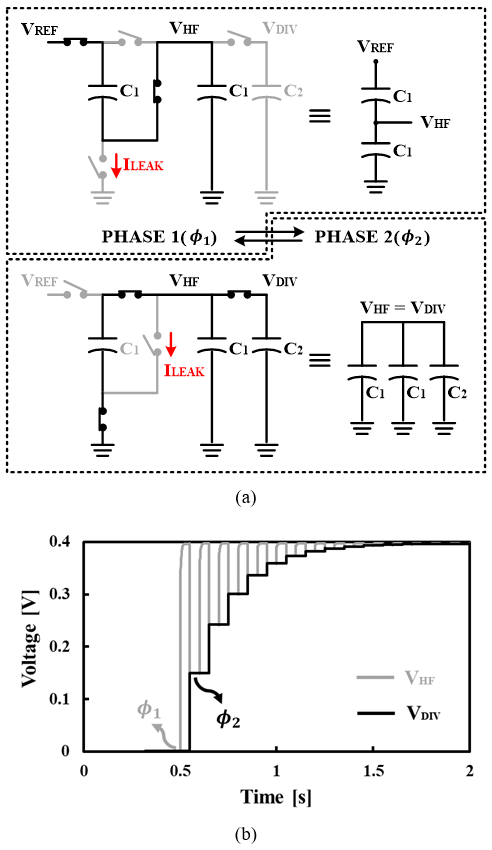The SC voltage divider is formed with three capacitors (Fig. 3(a)). They are two identical capacitors (C$_{1}$) of 2.5~pF and a larger storage capacitor (C$_{2}$) of 10 pF. The voltage division is performed using two phases, a receiving phase (${Φ}$$_{1}) and a transferring phase ({Φ}$$_{2}$). In ${Φ}$$_{1}, the two identical capacitors (C_{1}) are connected in series to sample V_{\mathrm{REF}} while C_{2} is disconnected. In {Φ}$$_{2}$, all the three capacitors are connected in parallel while V$_{\mathrm{REF}}$ is disconnected. Repetition of switching eventually makes C$_{2}$ hold V$_{\mathrm{REF}}$/2. Simulated transients of V$_{\mathrm{HF}}$ and V$_{\mathrm{DIV}}$ are shown in Fig. 3(b). In the steady state, the voltage stored at all the three capacitors approaches V$_{\mathrm{REF}}$/2, and there would be no charge transferring among the capacitors. Since the leakage current (I$_{\mathrm{leak}}$) through off-state switch (Fig. 3(a)) has a strong temperature dependency, the V$_{\mathrm{HF}}$ at high temperature significantly decays by the leakage. We adopted a switch in (15) which minimizes the effect of leakage current.

In addition, frequency for the switching needs to be designed so that the leakage current can be compensated to maintain V$_{\mathrm{REF}}$/2. We employed a leakage-based oscillator as the clock generator (Fig. 4). Designed parameters are summarized in Table 1. Bold characters indicate thick MOSFET. A capacitor of 30 pF is used for C$_{\mathrm{OSC}}$.

## III. MEASUREMENT RESULTS

The proposed temperature sensor is implemented using a standard 180 nm CMOS process. Fig. 5 shows chip microphotograph. The active die area is 0.128 mm$^{2}$. 37 chips are tested in a temperature range of -30~100 $^{\circ}$C. Fig. 6 shows measurements of the internally generated voltages which are V$_{\mathrm{REF}}$, V$_{\mathrm{CTAT}}$ and AVDD. The V$_{\mathrm{PTAT}}$ is calculated by V$_{\mathrm{REF}}$-V$_{\mathrm{CTAT}}$. The V$_{\mathrm{REF}}$ and AVDD are about 0.8 V and 0.4 V, respectively, showing a good agreement with designed levels. Fig. 7 shows digital codes measured from 37 samples. The dynamic range of the temperature-dependent voltage is about 150 mV (see Fig. 6) which corresponds to about 35% of AVDD. Thus, the range of the output code is also about 35% (from 250 to 600) of the total 10-b code range.

Table 1. Design parameters.

 Component Parameters Width (μm) Length (μm) Multiplier M$_{\mathrm{N1}}$ 0.42 1.2 1 M$_{1}$ 20 1 7 M$_{2}$ 1 1 1 M$_{\mathbf{PASS}}$ 0.5 0.85 1 M$_{\mathbf{P}}$ 5 5 5* M$_{\mathbf{N}}$ 4 10 5* M$_{\mathbf{B}}$ 1 1 12* M$_{\mathbf{O}}$ 20 1 21 M$_{\mathbf{DIS}}$ 1 0.35 3* Q$_{1}$ 2 2 2

* The number of stacks

Fig. 6. Measured internal voltages of bias generator. The V$_{\mathrm{PTAT}}$ is calculated by subtracting V$_{\mathrm{CTAT}}$ from V$_{\mathrm{REF}}$.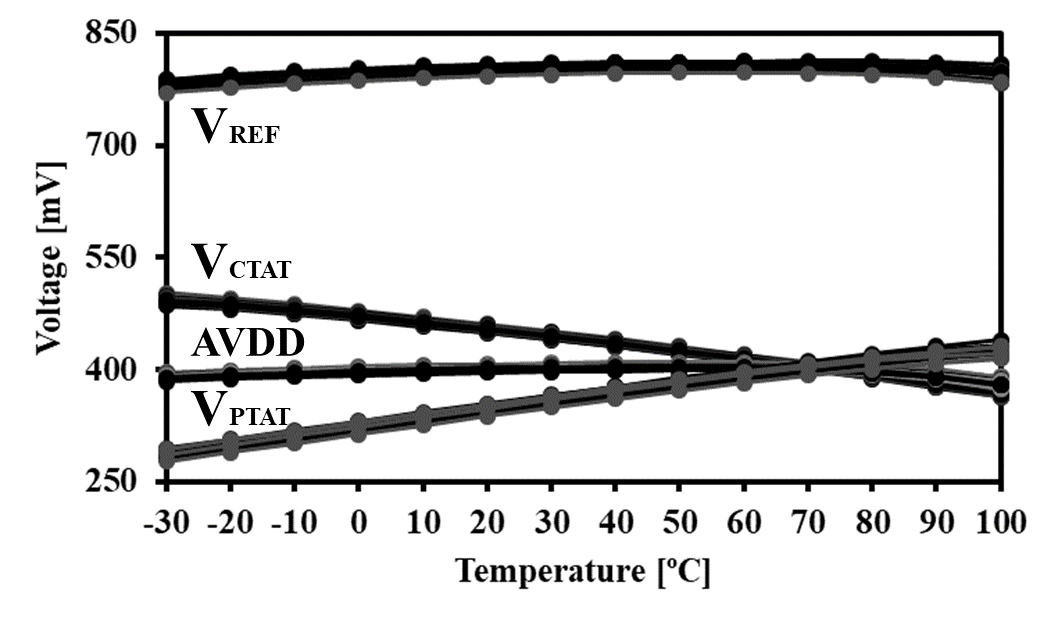Fig. 8. Error temperature after a 1-point calibration at 40 $^{\circ}$C calculated with the measured bias voltages and an ideal ADC.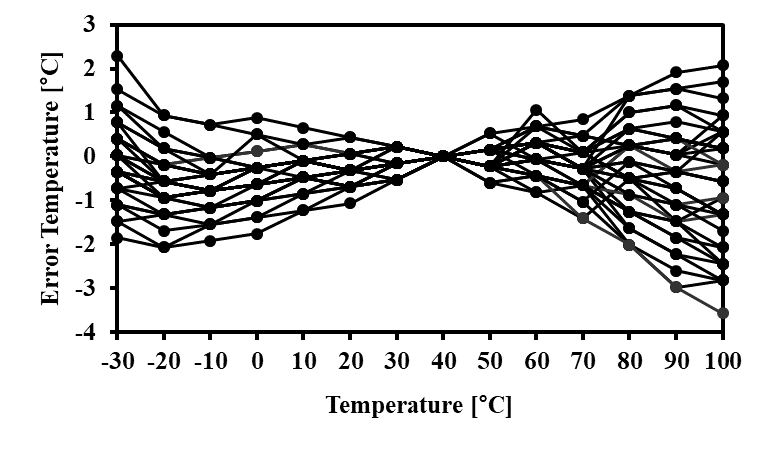Fig. 9. Measured error temperature after a 1-point calibration at 40 $^{\circ}$C.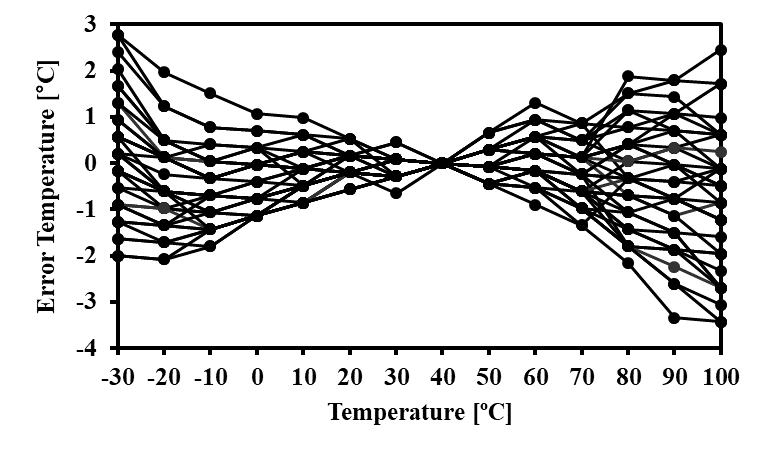To exclude imperfections of ADC operation in temperature sensing, error temperatures are calculated from the measured bias voltages (Fig. 6). An ideal ADC is modeled by MATLAB for the calculation. We compared the calculated error with the measured error using the implemented ADC. Fig. 8 and 9 show the calculated and the measured error temperature after a 1-point calibration at 40 $^{\circ}$C, respectively. The two figures indicate an overall good agreement with an increased error of about 1-LSB by ADC nonlinearity. The ADC simulation shows an input-referred comparator noise of about 550 ${μ}$Vrms which corresponds to 1.37-LSB of the ADC with an AVDD of 0.4 V. The capacitor DACs were implemented by a MIM structure with the unit C of 5 fF, indicating a 12-b level matching according to given 0.18~${μ}$m CMOS process documents. Therefore, the inaccuracy of temperature sensing is rather limited by the temperature dependences of the bias voltages than by the linearity performance of ADC.

Fig. 10. Measured error temperature after a 2-point calibration at -10 $^{\circ}$C and 80 $^{\circ}$C.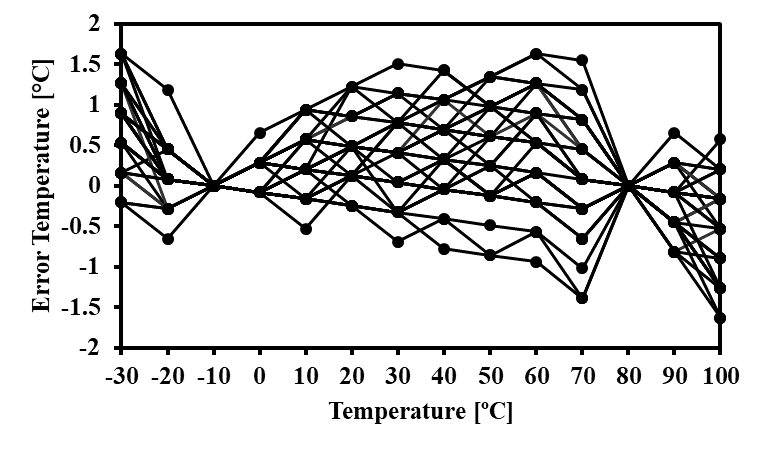Fig. 11. Measured oscillator frequency from 37 samples over a temperature range of -30-to-100 $^{\circ}$C.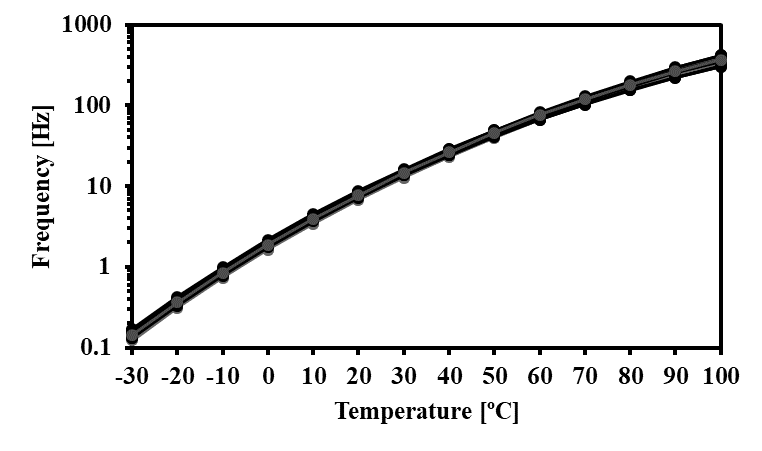Fig. 12. Measured RMS resolution (1-${\upsigma}$) after 500 sensor readings from each of 37 samples.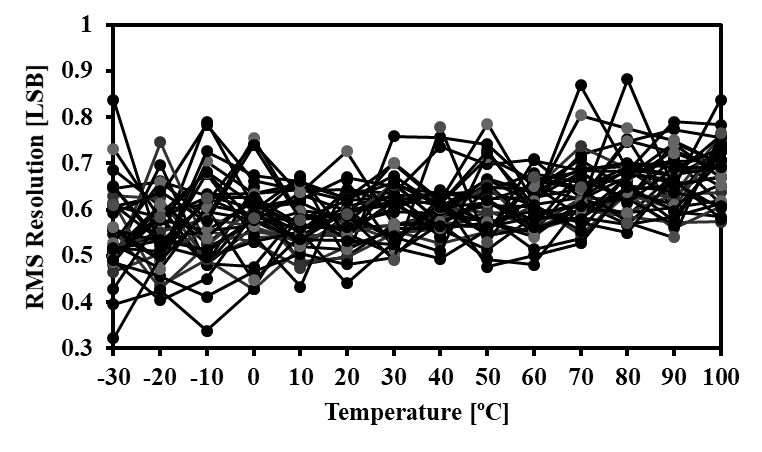Fig. 13. Measured supply sensitivities when the supply voltage varies from 1 V to 2.2 V.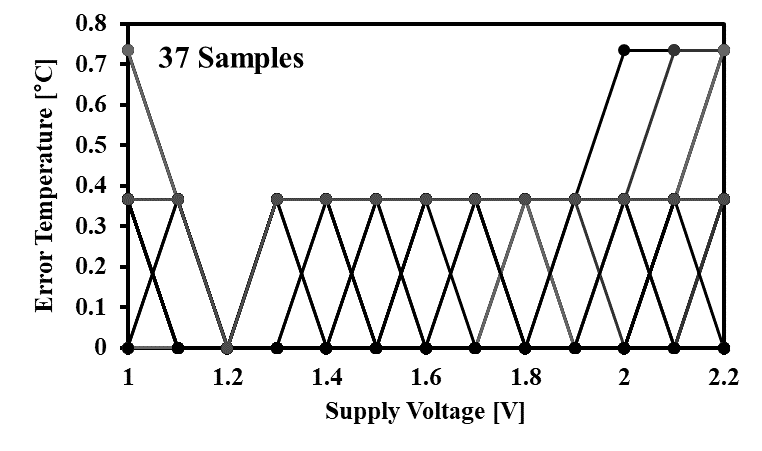Fig. 14. Average power consumption of each core block and the energy/conversion.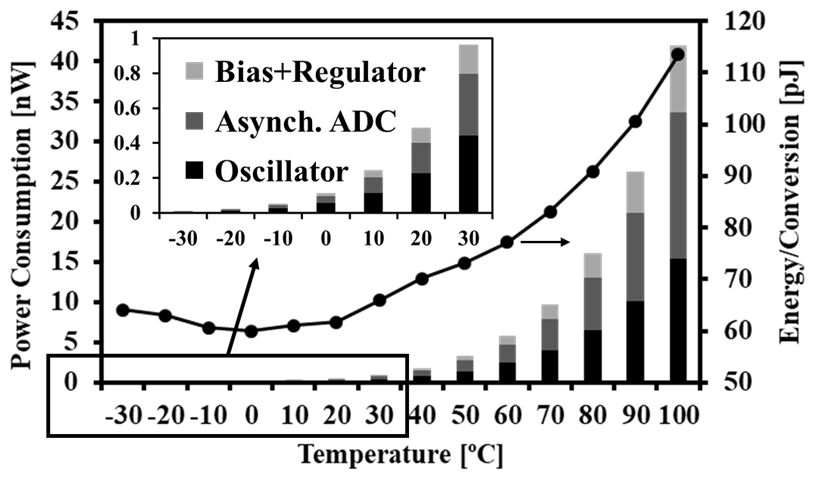A 2-point calibration at -10 $^{\circ}$C and 80 $^{\circ}$C reduces the peak-to-peak inaccuracy from -3.43 $^{\circ}$C/+2.77 $^{\circ}$C to -1.63~$^{\circ}$C/+1.63~$^{\circ}$C (Fig. 10). Fig. 11 shows measured frequency of the oscillator. The strong dependence of the oscillator frequency helps suppression of the effect of leakage current on the operation of the SC voltage divider. Fig. 12 shows the RMS resolutions (16) of 500 cases. The RMS resolution refers to the standard deviation of the converted temperature when the test temperature is fixed. The RMS resolution for the whole temperature range is less than 1-LSB, also revealing that the resolution of the sensor is rather limited by the quantized 1-b temperature resolution of 0.37 $^{\circ}$C. Fig. 13 shows measured supply sensitivity at 20 $^{\circ}$C, showing 0.61 $^{\circ}$C/V in a supply range of 1 V-to-2.2 V. By placing a stacked native NMOS transistors at the header of the bias generator, the effect of supply variation is greatly suppressed. Fig. 14 summarizes average power consumption and energy/conversion. The average power consumption at 20~$^{\circ}$C is 487 pW, indicating an energy/conversion of 62.6 pJ. The energy/conversion in the whole temperature range varies from 60 pJ to 110 pJ. Table 2 compares performance with previously reported low-power temperature sensors.

Table 2. Performance comparison

 This work       Process (nm) 180 180 180 180 65 180 180 Supply Voltage (V) 1.2 1 1.6~2.2 1 0.5, 0.9~1.2+ 0.8 0.6~1.2 Type PNP & MOS NPN & MOS NPN PNP MOS (Gate Leak.) MOS MOS Digital Conversion SAR SAR ΣΔ ΣΔ FDC FDC FDC Supply Sensitivity (°C/V) 0.61 1.073 0.0082 N/A N/A 3.8 1.9 Power (nW) 0.487 0.49 8960 720 0.64 11 3.92 Conversion Time (ms) 128.6 200 213 40 34.3 839 300 Energy/Conversion (nJ) 0.0626 0.098 1908.5 28.8 0.022 9.2 1.2 Temperature Range (°C) -30~100 -10~100 -40~125 0~100 -20~100 -20~80 0~100 Resolution (K) 0.37 0.59 0.00167 0.04 0.25 0.145 0.55 Calibration 1-point 2-point 1-point 1-point 1-point 2-point 2-point 2-point Inaccuracy (°C) -3.43/2.77* (37 smps) ±1.63* (37 smps) ±2.35* (15 smps) ±0.13** (16 smps) ±0.18** (15 smps) -2.7/1.8* (7 smps) -0.9/1.2* (9 smps) -1.64/0.67* (3 smps) Area (mm$^{2}$) 0.1278 0.12 0.35 0.18 0.013 0.074 0.007 FoM$_1$ (pJK$^{2}$)# 8.57 34.1 5.32 46.08 1.4 193.4 363 FoM$_2$ (nJ°C$^{2}$)## 0.74 0.17 0.54 32.25 0.93 0.16 13.25 3.23

+ : two supply voltages

* : peak-to-peak inaccuracy

** : 3σ inaccuracy

# : FoM$_{1}$ = energy/conversion ${\times}$ (resolution)$^{2}$

## FoM$_{2}$ = energy/conversion ${\times}$ (max inaccuracy)$^{2}$

## IV. CONCLUSIONS

This work proposes a leakage-based temperature sensor with a SC voltage divider. The temperature sensor consists of a temperature sensing part and an asynchronous SAR ADC. The SC voltage divider performs a division of V$_{\mathrm{REF}}$ by half to provide supply voltage for ADC. It effectively achieves a finer temperature resolution with given ADC. The proposed temperature sensor chip is fabricated in an active area of 0.1278 mm$^{2}$ using 180 nm CMOS technology. The implemented sensor consumes 487 pW with an energy/conversion of 62.6 pJ. The proposed work minimally dissipates standby power for analog circuits and is promising for ULP sensor applications which necessitate continuous thermal monitoring.

### REFERENCES

1
Kumar Rushil K., Nov 2019, An Energy-Efficient BJT-Based Temperature-to-Digital Converter with ±0.13ºC (3σ) Inaccuracy from -40 to 125ºC, IEEE ASSCC, pp. 107-1082
Zhan Tan-Tan, Apr 2018, Nano-Watt Class Energy-Efficient Capacitive Sensor Interface With On-Chip Temperature Drift Compensation, IEEE Sensors Journal, Vol. 18, No. 7, pp. 2870-28823
Tang Zhong, Nov 2018, A CMOS Temperature Sensor With Versatile Readout Scheme and High Accuracy for Multi-Sensor Systems, IEEE Trans. Circuits Syst. I, Vol. 65, No. 11, pp. 3821-38294
Yousefzadeh B., Jun 2016, A BJT-based Temperature-to-Digital Converter with ±60mK (3σ) Inaccuracy from -70ºC to 125ºC in 160nm CMOS, IEEE Symp. VLSI Circuits5
Oshita Takao, Mar 2015, Compact BJT-Based Thermal Sensor for Processor Applications in a 14nm tri-Gate CMOS Process, IEEE JSSC, Vol. 50, No. 3, pp. 799-8076
Truesdell Daniel S., Apr 2019, A 640pW 22pJ/Sample Gate Leakage-Based Digital CMOS Temperature Sensor with 0.25ºC Resolution, CICC, pp. 1-47
Someya Teruki, Mar 2019, An 11-nW CMOS Temperature-to-Digital Converter Utilizing Sub-Threshold Current at Sub-Thermal Drain Voltage, IEEE JSSC, Vol. 54, No. 2, pp. 613-6228
Ku Chia-Yuan, Oct 2019, A Voltage-Scalable Low-Power All-Digital Temperature Sensor for On-Chip Thermal Monitoring, IEEE Trans. Circuits Syst. II, Vol. 66, No. 10, pp. 1658-16629
Y. K., Feb 2017, A 0.6nJ -0.22/0.19ºC Inaccuracy Temperature Sensor Using Exponential Subthreshold Oscillation Dependence, IEEE ISSCC, pp. 160-16210
Anand Tejasvi, Jun 2015, A Self-referenced VCO-based Temperature Sensor with 0.034ºC/mV Supply Sensitivity in 65nm CMOS, IEEE Symp. VLSI Circuits11
Islam A. K. M. Mahfuzul, Nov 2015, Wide-Supply-Range All-Digital Leakage Variation Sensor for On-Chip Process and Temperature Monitoring, IEEE JSSC, Vol. 50, No. 11, pp. 2475-249012
Jeong Seokhyeon, Aug 2014, A Fully-Integrated 71nW CMOS Temperature Sensor for Low Power Wireless Sensor Nodes, IEEE Journal of Solid-State Circuits, Vol. 49, No. 8, pp. 1682-169313
Tang Zhong, Nov 2018, A CMOS Temperature Sensor With Versatile Readout Scheme and High Accuracy for Multi-Sensor Systems, IEEE Trans. Circuits Syst. I, Vol. 65, No. 11, pp. 3821-382914
Ji Youngwoo, Feb 2019, A 192pW Hybrid Bandgap-Vth Reference with Process Dependence Compensated by a Dimension-Induced Side-Effect, in IEEE Int. Solid-State Circuits Conf. (ISSCC), pp. 308-31015
Ishida K., Arl 2006, Managing Subthreshold Leakage in Charge-Based Analog Circuits With Low-VTH Transistors by Analog T- Switch (AT-Switch) and Super Cut-off CMOS (SCCMOS), IEEE JSSC, Vol. 41, No. 4, pp. 859-86716
Makinwa K.A.A., Delft Tu, Smart Temperature Sensor Survey, Rev. 04022020, Arl. 2020. Accessed on Dec. 24, 2020. https://ei.tudelft.nl/smart_temperature/## Author

Bumjin Park received the B.S. and M.S. degrees in Electronic and Electrical Engineering from Pohang University of Science and Technology, South Korea, in 2014, and 2016, respectively.

He is currently pursuing the Ph.D. degree in the Department of Electronic and Electrical Engineering from Pohang University of Science and Technology (POSTECH), Korea.

His interests include and ultra-low-power sensor interface circuits

Youngwoo Ji received the B.S. and Ph.D. degrees in electronic and electrical engineering from the Pohang University of Science and Technology (POSTECH), Pohang, South Korea, in 2013 and 2020, respectively.

He is currently a Post-Doctoral Researcher with the Integrated Systems Laboratory, ETH Zürich, Zürich, Switzerland.

His research interests include subthreshold circuit designs, sensor interface circuits, and data converters.

Jae-Yoon Sim received the B.S., M.S., and Ph.D. degrees in electronic and electrical engineering from the Pohang University of Science and Technology (POSTECH), South Korea, in 1993, 1995, and 1999, respectively.,

From 1999 to 2005, he was a Senior Engineer with Samsung Electronics, South Korea.

From 2003 to 2005, he was a Postdoctoral Researcher with the University of Southern California, Los Angeles.

From 2011 to 2012, he was a Visiting Scholar with the University of Michigan, Ann Arbor.

In 2005, he joined the Department of Electrical Engineering, POSTECH, where he is currently a Professor.

His research interests include serial and parallel links, PLLs, data converters, ultra-low-power sensor circuits, and power module for plasma generation.

Dr. Sim received the Special Author-Recognition Award at ISSCC 2013 and was a co-recipient of the Takuo Sugano Award at ISSCC 2001.

He has served on the Technical Program Committee of the IEEE International Solid-State Circuits Conference (ISSCC), the Symposium on VLSI Circuits, and the Asian Solid-State Circuits Conference (ASSCC).